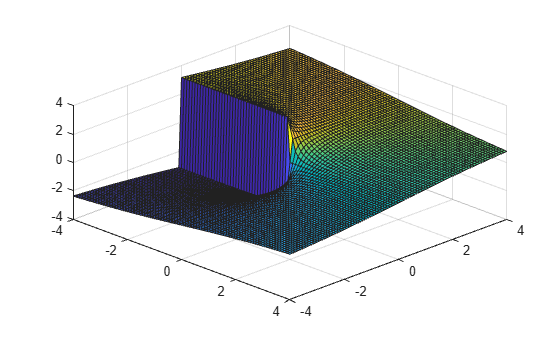Documentation

# atan2

## Syntax

``P = atan2(Y,X)``

## Description

example

````P = atan2(Y,X)` returns the four-quadrant inverse tangent (tan-1) of `Y` and `X`, which must be real. The `atan2` function follows the convention that `atan2(x,x)` returns `0` when `x` is mathematically zero (either `0` or `-0`).```

## Examples

collapse all

Find the four-quadrant inverse tangent of the point `y = 4`, `x = -3`.

`atan2(4,-3)`
```ans = 2.2143 ```

Convert `4 + 3i` into polar coordinates.

```z = 4 + 3i; r = abs(z)```
```r = 5 ```
`theta = atan2(imag(z),real(z))`
```theta = 0.6435 ```

The radius `r` and the angle `theta` are the polar coordinate representation of `4 + 3i`.

Alternatively, use `angle` to calculate `theta`.

`theta = angle(z)`
```theta = 0.6435 ```

Convert `r` and `theta` back into the original complex number.

`z = r*exp(i*theta)`
```z = 4.0000 + 3.0000i ```

Plot `atan2(Y,X)` for `-4<Y<4` and `-4<X<4`.

Define the interval to plot over.

`[X,Y] = meshgrid(-4:0.1:4,-4:0.1:4);`

Find `atan2(Y,X)` over the interval.

`P = atan2(Y,X);`

Use `surf` to generate a surface plot of the function. Note that `plot` plots the discontinuity that exists at `Y=0` for all `X<0`.

```surf(X,Y,P); view(45,45);```## Input Arguments

collapse all

y-coordinates, specified as a scalar, vector, matrix, or multidimensional array. Inputs `Y` and `X` must either be the same size or have sizes that are compatible (for example, `Y` is an `M`-by-`N` matrix and `X` is a scalar or `1`-by-`N` row vector). For more information, see Compatible Array Sizes for Basic Operations.

Data Types: `single` | `double`

x-coordinates, specified as a scalar, vector, matrix, or multidimensional array. Inputs `Y` and `X` must either be the same size or have sizes that are compatible (for example, `Y` is an `M`-by-`N` matrix and `X` is a scalar or `1`-by-`N` row vector). For more information, see Compatible Array Sizes for Basic Operations.

Data Types: `single` | `double`

collapse all

The four-quadrant inverse tangent, `atan2(Y,X)`, returns values in the closed interval `[-pi,pi]` based on the values of `Y` and `X`, as shown in the graphic.In contrast, `atan(Y/X)` returns results that are limited to the interval `[-pi/2,pi/2]`, shown on the right side of the diagram.

### IEEE Compliance

For real inputs, `atan2` has a few behaviors that differ from those recommended in the IEEE®-754 Standard.

MATLAB® IEEE
`atan2(0,-0)`

`0`

`pi`

`atan2(-0,-0)`

`0`

`-pi`